ď»ż

### Introduzione

#### Abstract

En
Some authors have recently introduced cohomological spaces related to a linear connection on a real differentiable manifold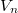$V_n$. In this paper a cohomological space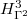$H_{\Gamma^2}^3$ related to a second-order connection of type (0,1)on$V_n$ and a sequence of cohomological spaces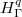$H_\Gamma^q$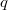$q$ in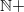$\mathbb{N}+$ related to a linear pseudoconnection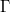$\Gamma$ of$V_n$ are introduced.Some essential properties of such spaces of cohomology are investigated an meaningfuld examples are provided.

DOI Code: §

Full Text: PDF